# Relationship Between A.M. & G.M. Notes | Study Business Mathematics and Logical Reasoning & Statistics - CA Foundation

## CA Foundation: Relationship Between A.M. & G.M. Notes | Study Business Mathematics and Logical Reasoning & Statistics - CA Foundation

The document Relationship Between A.M. & G.M. Notes | Study Business Mathematics and Logical Reasoning & Statistics - CA Foundation is a part of the CA Foundation Course Business Mathematics and Logical Reasoning & Statistics.
All you need of CA Foundation at this link: CA Foundation

Let a and b be the two numbers.

Let A and G be the A. M. and G. M. respectively between a and b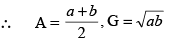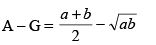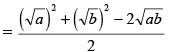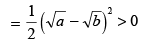∴ A > G

Example 1. The arithemetic mean between two numbers is 34 and their geometric meanis 16. Find the numbers.

Solution : Let the numbers be a and b.

Since A. M. between a and b is 34,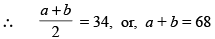.....(1)

Since G. M. between a and b is 16,

∴  √ab = 16 or, ab = 256

we know that  (a – b)2 = (a + b)2 – 4 ab ......(2)

= (68)2 – 4 × 256
= 4624 – 1024 = 3600

∴    a – b = √3600 = 60 ....... (3)

Adding (1) and (3), we get, 2a = 128

∴ a = 64

Subtracting (3) from (1), are get

2b = 8    or, b = 4

∴ Required numbers are 64 and 4.

Example 2. The arithmetic mean between two quantities b and c is a and the twogeometric means between them are g1 and g2. Prove that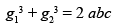Solutions: The A. M. between b and c is a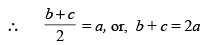Again g1 and g2 are two G. M.'s between b and c

∴ b, g1, g2, c are in G. P.

If r be the common ratio, then

c = br3

or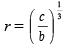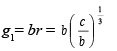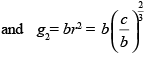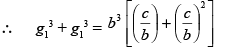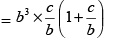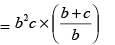= bc (2a)  [since b + c = 2a]

= 2abc

Example 3. If one geometric mean G and two arithmetic means p and q be inserted between two quantities, show that : G2 = (2p – q) (2q – p)

Solutions: Let the two quantities be a and b, then

G = √ab or, G2 = ab ...... (1)

Also p and q are two A. M.'s betweena and b

∴ a, p, q, b are in A. P.

∴ p – a = q – p and q – p = b – q

∴ a = 2p – q and b = 2q – p

∴ G2 = ab = (2p – q) (2q – p)

Example 4. The product of first three terms of a G. P. is 1000. If we add 6 to its secondterm and 7 to its 3rd term, the three terms form an A. P. Find the terms of the G. P.

Solutions: Let t1 = a/r, t2 = a and t3 = ar be the first three terms of G. P.

Then, their product = a/r. a.ar = 1000 or a3 = 1000 or a = 10

By the question, t1, t2 + 6, t3 + 7 are in A. P.   ...... (1)

i.e, a/r, a + 6, ar + 7 are in A.P.

∴ (a + 6) – a/r  = (ar + 7) – (a + 6)

or (a + 6)  = a/r +  (ar + 7)

or, 2(10+6) = 10/r + (10r + 7)

or, 32r = 10 + 10 r2 + 7r

or, 10r2 – 25r + 10 = 0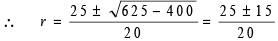= 2, 1/2

When a = 10, r = 2. then the terms are 10/2, 10(2) i.e. 5,10,20

When a = 10, r =  1/2, then the terms are 10(2), 10, 10(1/2)

i.e., 20, 10, 5

The document Relationship Between A.M. & G.M. Notes | Study Business Mathematics and Logical Reasoning & Statistics - CA Foundation is a part of the CA Foundation Course Business Mathematics and Logical Reasoning & Statistics.
All you need of CA Foundation at this link: CA FoundationUse Code STAYHOME200 and get INR 200 additional OFF

## Business Mathematics and Logical Reasoning & Statistics

81 videos|101 docs|87 tests

Track your progress, build streaks, highlight & save important lessons and more!

,

,

,

,

,

,

,

,

,

,

,

,

,

,

,

,

,

,

,

,

,

;